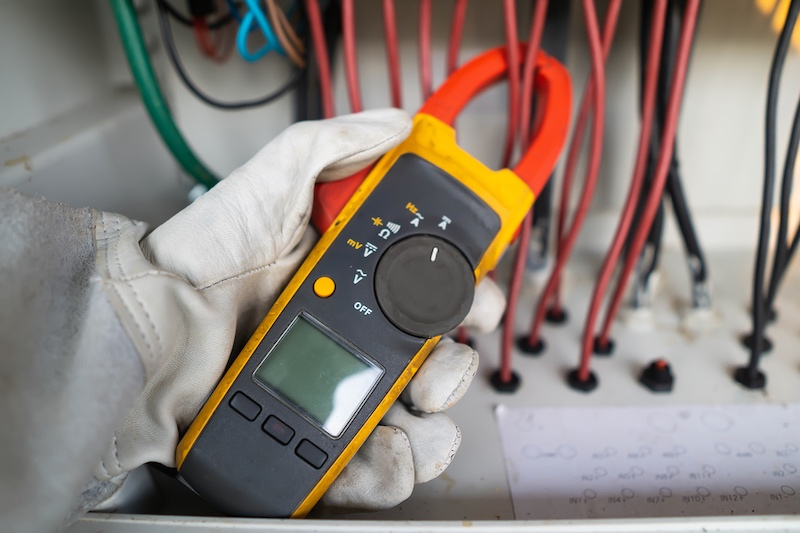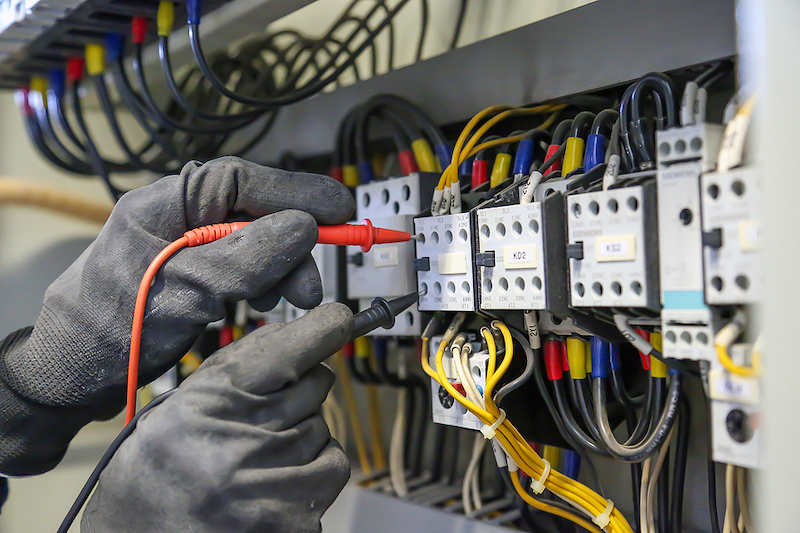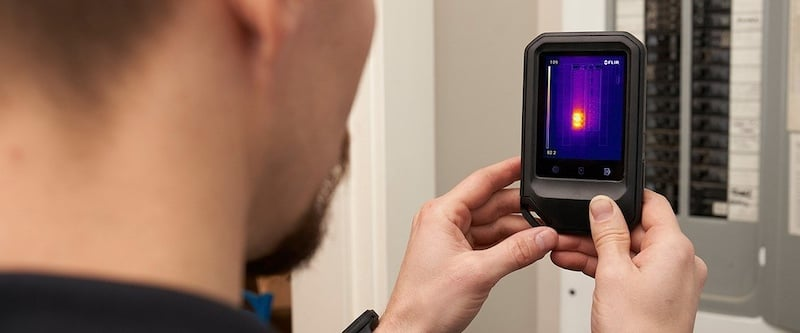Technical Article

# Common Mathematical Units for Electrical Troubleshooting and Control Operations

May 10, 2021 by David Peterson

## In industrial control, it is crucial to have a basic understanding of the units that define and describe the ratings of components.

The subject of mathematics is far too broad to assume that every concept will apply equally to every situation and industry. A previous article discusses the importance of mathematics for troubleshooting and control.

Some jobs will require knowledge of calculus and differential equations to solve complex motion situations. However, in more common day-to-day tasks for electrical troubleshooting, it is critical to understand the relationships between electrical quantities and measurements.

This involves a basic understanding of the units that define and describe the ratings of components. This article provides an overview of some mathematical units that industrial engineers and technicians may need to understand in their daily operations.

### An Overview of Mathematical Units

In the field of industrial and electrical troubleshooting, it’s difficult to imagine a single situation in which the terms voltage, power, or current do not make some sort of appearance. It’s easy to skim over these values or to make measurements without fully understanding why they exist.##### A multimeter being used in an industrial environment to measure current, voltage, and other mathematical units.

That’s fine for many situations, but it adds a new level of troubleshooting ability to fully realize how the different values are connected and why a change of one unit measurement could affect an entire system. Tracing problems to a root cause always requires the ability to understand the cascading effects of problems leading to certain symptoms further in the process.

#### Voltage

The ‘volt’ is perhaps the most commonly used electrical term, perhaps with the exception of power units. The volt is described most simply as the driving force that can push electron motion as long as a path is provided. Formally, voltage is defined as the energy (joules) required to drive one coulomb of charge from one point to another.

If voltage is increased, it could be expected that more coulombs of charge would move, leading to greater current (see below). In relation to resistance, if a circuit has increased resistance to electron flow, the voltage must be increased to maintain the proper amount of current.

#### Current

Current is the result of electrons in motion, called ‘amps.’ This is in contrast to voltage since voltage may be present even if no electron motion is happening. Current is almost always the result of other circuit properties - an improper current should be traced back to how the current changed.

There are only two values that can affect current. However, there are unlimited situations that can cause these other two values to change. As described above, voltage adjustments will cause a similar change to current. If a voltage source is lowered, expect the current to become lower. If the voltage is increased, the current will also increase, which often causes overheating and damage to the circuit. Always avoid over-voltage.##### An engineer testing the power and current within a facility.

Resistance also affects current but in an inverse manner. If resistance is increased, even up to an infinite ‘open circuit’ resistance, the current will decrease to the point of zero.

Solid-state components are designed to exhibit high resistances while still performing their functions, leading to very low current circuits, which save power. Low resistances such as in wires, conductors, or large motor coils lead to high current values.

#### Power

In most everyday situations, two references to power are very common. In mechanical power systems, the term ‘horsepower’ refers to the output of an engine or motor. It could also be the power required to drive systems with gears, belts, chains, and other components.

The other common term for power is the ‘watt,’ usually reserved for electrical power measurements. The watt is equal to one volt driving one amp of current through a point in a wire. However, since the volt indicates energy, and the current indicates motion, the watt can also be expressed as the change of one Joule of energy from one form to another in one second. For reference, 1 horsepower is roughly equal to 750 watts.

The goal of any circuit is to accomplish work, and this conversion of work (or energy) from one type to another is perhaps the most important property that can be expressed about any circuit. Power can originate as a chemical or mechanical generator like a battery, solar panel, or hydroelectric source. It can then be converted back into light, heat, sound, motion, or whatever other task is required of a circuit.

Power is not an accumulated value. You cannot express how much power is used during a day, or a month, etc. You can only express power as an instantaneous value - how fast is energy being used or converted right at this moment? For the total cumulative value, see ‘energy’ later in the article.

#### Heat Dissipation

Heat dissipation is really nothing more than a specialized form of power. However, it can be one of the most critical forms of power because it can be used for good use. It can present problems, but heat is almost always present in every live circuit.

Heat dissipation, expressed in watts, results anytime there is current traveling through any sort of resistance that causes a drop in voltage. This is commonly seen in load devices since they exist solely to convert energy into practical uses.##### A thermal camera detecting heat in an industrial wiring panel. Image used courtesy of FLIR

Heat loss is also common in wires that are too small, connection points that are loose or damaged. Solid-state switching devices can be efficient but still have some tiny amount of internal resistance. The goal of nearly every wiring or troubleshooting method is to reduce heat losses and increase the efficiency of a circuit. This can save the power for where it is most needed-at the load device.

A follow-up article will discuss more electrical units used in troubleshooting techniques, including the following:

• Resistance
• Impedance
• Energy
• Arc Flash Energy
• Frequency

The following article will dive more into some of those additional units that engineers and technicians may need to know.